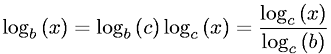Equations > Algebra > Logarithms > Logarithm Base Conversion

### Logarithm Base ConversionLatex Code:

MathML Code:

 ${\mathrm{log}}_{b}\left(x\right)={\mathrm{log}}_{b}\left(c\right){\mathrm{log}}_{c}\left(x\right)=\frac{\mathrm{log}}_{c}\left(x\right){\mathrm{log}}_{c}\left(b\right)$

MathType 5.0: